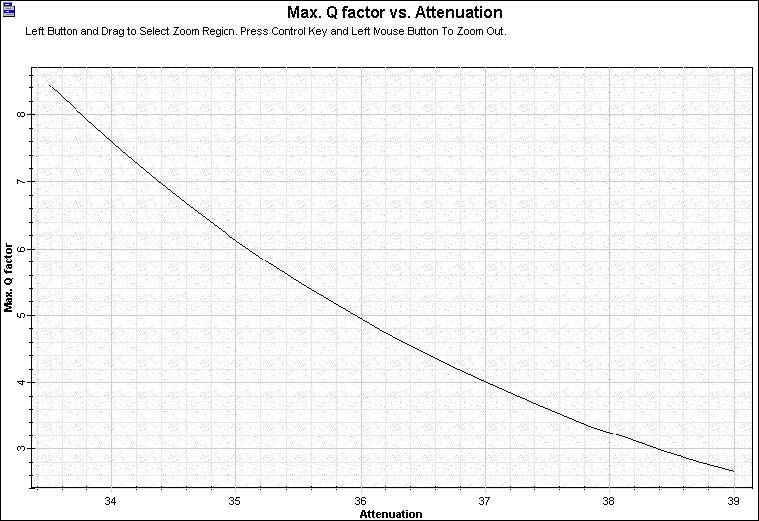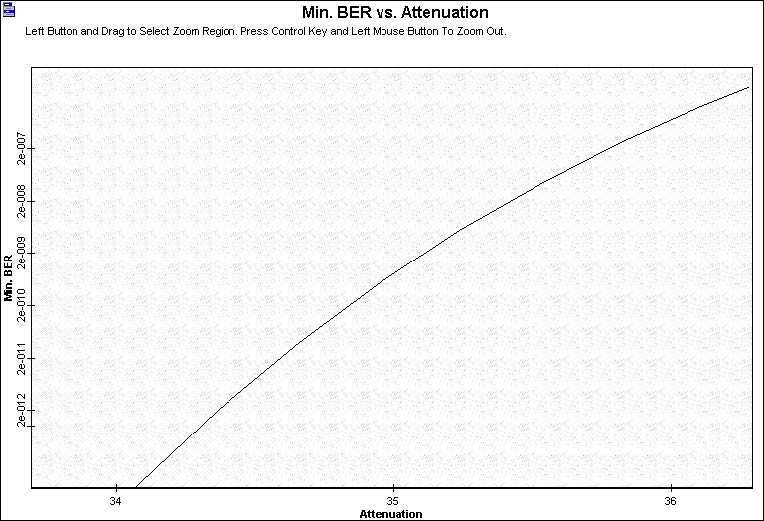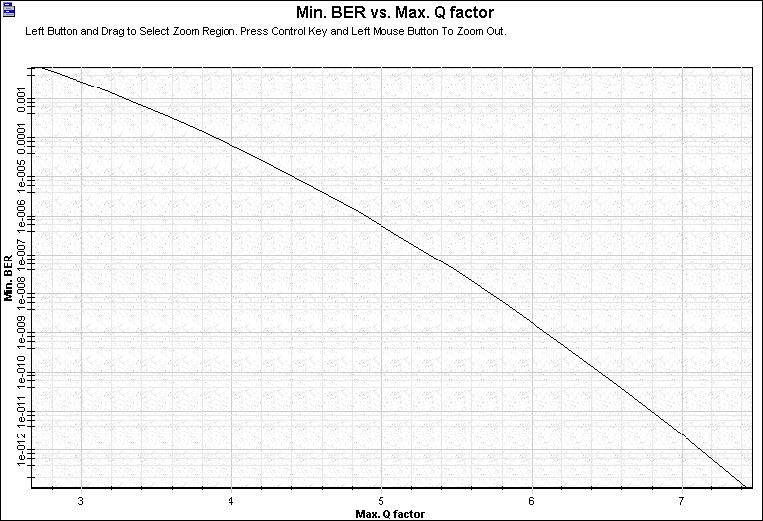The performance criteria for digital receivers if governed by the bit-error-rate (BER), defined as the probability of incorrect identification of a bit by the decision circuit of the receiver. Receiver BER – Q factor.osd shows the BER and Q factor at the data recovery stage for different values of input power.

## Receiver BER – Q factor

If you change the signal input power, you can calculate Q Factor and BER versus attenuation, in addition to BER versus Q factor (see Figure 1 for Maximum Q factor vs. Attenuation, Figure 2 for Minimum BER vs. Attenuation, and Figure 3 for minimum BER vs. Maximum Q factor).Figure 1: Q Factor x AttenuationFigure 2: BER x AttenuationFigure 3: BER x Q Factor